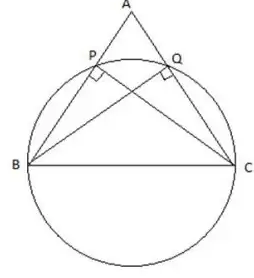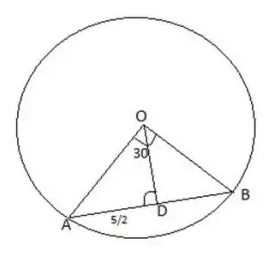### CAT 2018 – Slot 2 – Quantitative Aptitude – On a triangle ABC, a circle with diameter BC is drawn

Q. 1: On a triangle ABC, a circle with diameter BC is drawn, intersecting AB and AC at points P and Q, respectively. If the lengths of AB, AC, and CP are 30 cm, 25 cm, and 20 cm respectively, then the length of BQ, in cm, isAs CP is perpendicular to AB and BQ is perpendicular to AC. So

AB×CP=AC×BQ

30×20=25×BQ

BQ = 24 cm

##### Q. 2: A chord of length 5 cm subtends an angle of 60° at the centre of a circle. The length, in cm, of a chord that subtends an angle of 120° at the centre of the same circle is1. 82. 6√23. 5√34. 2In triangle ODA, OA=AD cosec 30=5
So if the angle AOB is 120 degree, then angle AOD will be 120/2=60
In the case length of chord = 2×OA Sin 60=5√3

Q. 3: Let f(x)=max{5x, 52-2x^2}, where x is any positive real number. Then the minimum possible value of f(x) is?

For f(x) to be minimum , 5 = 52 − 2^2

2^2+5 −52 =0

−42+13=0

= 4

Thus minimum value of f(x) = 5x = 5*4 = 20

Q. 4: A 20% ethanol solution is mixed with another ethanol solution, say, S of unknown concentration in the proportion 1:3 by volume. This mixture is then mixed with an equal volume of 20% ethanol solution. If the resultant mixture is a 31.25% ethanol solution, then the unknown concentration of S is
1. 52%
2. 50%
3. 55%
4. 48%

Let the volume of 20% ethanol solution in first mixtures = x so

Volume of S = 3x

Total volume of first solution = 4x

Total volume of final solution =2×4x=8x

Volume of ethanol solution in final mixture = x + 4x = 5x ,

So volume of S in final solution = 3x

Thus (31.25-20)/(s-31.25) = 3x/5x = 3/5

56.25=3s-93.75

3s=150

s=50%

Q. 5: A tank is emptied everyday at a fixed time point. Immediately thereafter, either pump A or pump B or both start working until the tank is full. On Monday, A alone completed ?lling the tank at 8 pm. On Tuesday, B alone completed filling the tank at 6 pm. On Wednesday, A alone worked till 5 pm, and then B worked alone from 5 pm to 7 pm, to fill the tank. At what time was the tank ?lled on Thursday if both pumps were used simultaneously all along?
1. 4:36 pm
2. 4:12 pm
3. 4:24 pm
4. 4:48 pm

Let pump A alone can fill the tank in t hours so time taken by pump B alone = t-2 hours

As per question , (t-3)/t+2/(t-2)=1

t^2-3t+6=t^2-2t

Or t=6

So the time at which pumps tank is emptied = 8 pm – 6 hours = 2 pm

Time taken by both pumps together to fill the tank =(6×4)/(6+4)=2.4 hours=2 hour 24 minutes

Thus tank will be filled by 2 pm + 2 hour 24 minutes=4:24 pm

##### Checkout Other Questions of CAT 2018 Slot 2 Paper:

Verbal Ability :              |   Q.01- Q.05  |  Q.06- Q.9  |  Q.10- Q.14  |  Q.15- Q.19  |  Q.20- Q.24  |  Q.25- Q.29  |  Q.30 – Q.34  |

Logical Reasoning :    |   Q.29 – Q.32  |

Quantitative Aptitude: |